# Cumulative Frequency

Cumulative Frequency

In this lesson, we will first understand the basics of cumulative frequency.

Consider this situation: dad has given you $5 as pocket money for the week. By the end of the week, you have$3. Dad gives you another $5 for the following week. This new weekly pocket money along with the$3 that you have saved accumilates to \$8

Still doubtful? Look at the example of Natalie below.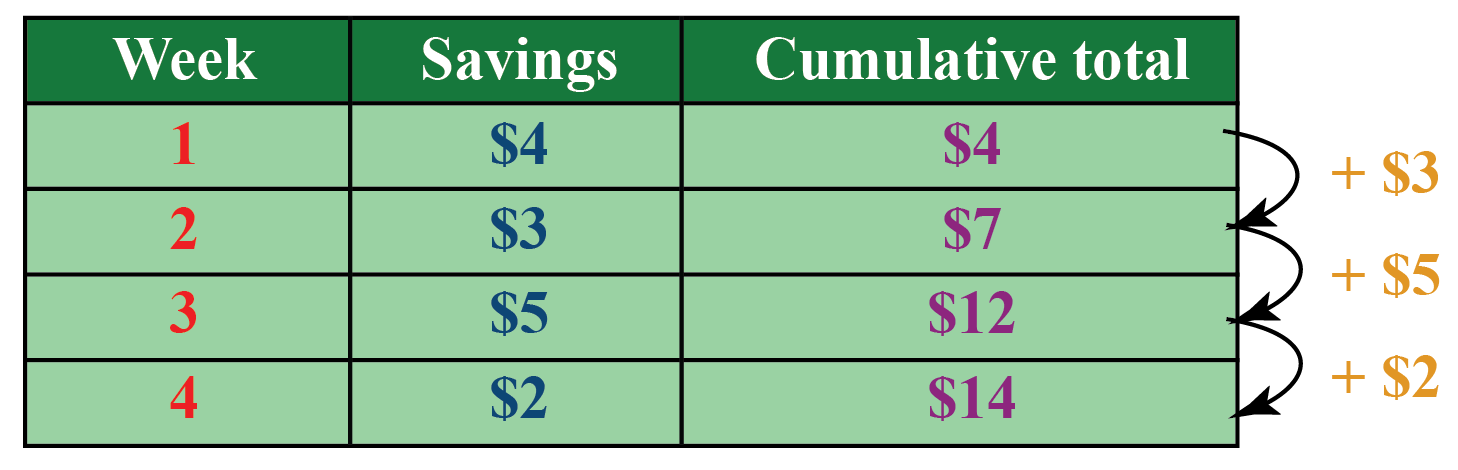Cumulative frequency is the total of a frequency and all frequencies in a frequency distribution until a certain defined class interval.

In this chapter, you will also learn how to plot a culumative frequency graph and learn how to read a cumulative frequency table.

Check out the interactive examples to know more about the lesson and try your hand at solving a few interesting practice questions at the end of the page.

## Lesson Plan

 1 What is Cumulative Frequency? 2 Thinking Out of the Box! 3 Important Notes on Cumulative Frequency 4 Solved Examples on Cumulative Frequency 5 Interactive Questions on Cumulative Frequency

## What is Cumulative Frequency?

In statistics, the frequency of the first-class interval is added to the frequency of the second class, and this sum is added to the third class and so on then, frequencies which are obtained this way are known as cumulative frequency (c.f.)

## Why is the Cumulative Frequency used?

You will find the use of cumulative frequencies in many real-world situations.

Let us see a couple of examples.

Example 1:

Robert is the sales manager of a toy company. On checking his quarterly sales record, he can observe that by the month of April, a total of 83 toy cars were sold.

Month Number of toy cars sold (Frequency) Total number of toy cars sold (Cumulative Frequency)
January 20 20
February 30 20 + 30 = 50
March 15 50 + 15 = 65
April 18 65 + 18 = 83

Note how the last cumulative total will always be equal to the total for all observations since all frequencies will already have been added to the previous total. Here, $$83 = 20 + 30 + 15 +18$$

Example 2:

A Major League Baseball team records its home-runs in the 2020 session as given below.Match f (home runs) cf (cumulative total)
Qualifying match 11 11
Quarterfinal match 8 11 + 8 = 19
Semifinal 10 19 + 10 = 29
Final 7 29 + 7 = 36

From the above table, it can be observed that the team made 29 home runs before playing the finals.

Thus, we can use the cumulative frequency to know the number of observations that lie above (or below) a particular frequency in a given data set.Think Tank
• A cumulative percentage is also known as a percentile rank. If you scored in the 85th percentile on the SAT, how many of the students taking the test had the same or a lower score than you?

## How to Make a Cumulative Frequency Distribution Table?

A table showing the cumulative frequencies is called a cumulative frequency distribution table.

Steps to make a cumulative frequency distribution table are as follows:

Step 1: Use the continuous variables to set up a frequency distribution table using a suitable class length.

Step 2: Find the frequency for each class interval.

Step 3: Locate the endpoint for each class interval (upper limit or lower limit).

Step 4: Calculate the cumulative frequency by adding the numbers in the frequency column.

Step 5: Record all results in the table.

We can also use this information gathered from the frequency distribution table to plot a cumulative frequency graph which we will learn a little later.

Example:

During a 20-day long skiing competition, the snow depth at Snow Mountain was measured (to the nearest cm) for each of the 20 days. The records are as follows:

301, 312, 319, 354, 359, 345, 348, 341, 347, 344, 349, 350, 325,323, 324, 328,322, 332, 334, 337#### Answer: Using the same steps mentioned above, a cumulative frequency distribution table can be made as:

Snow depth measured at Snow Mountain, the 20-day period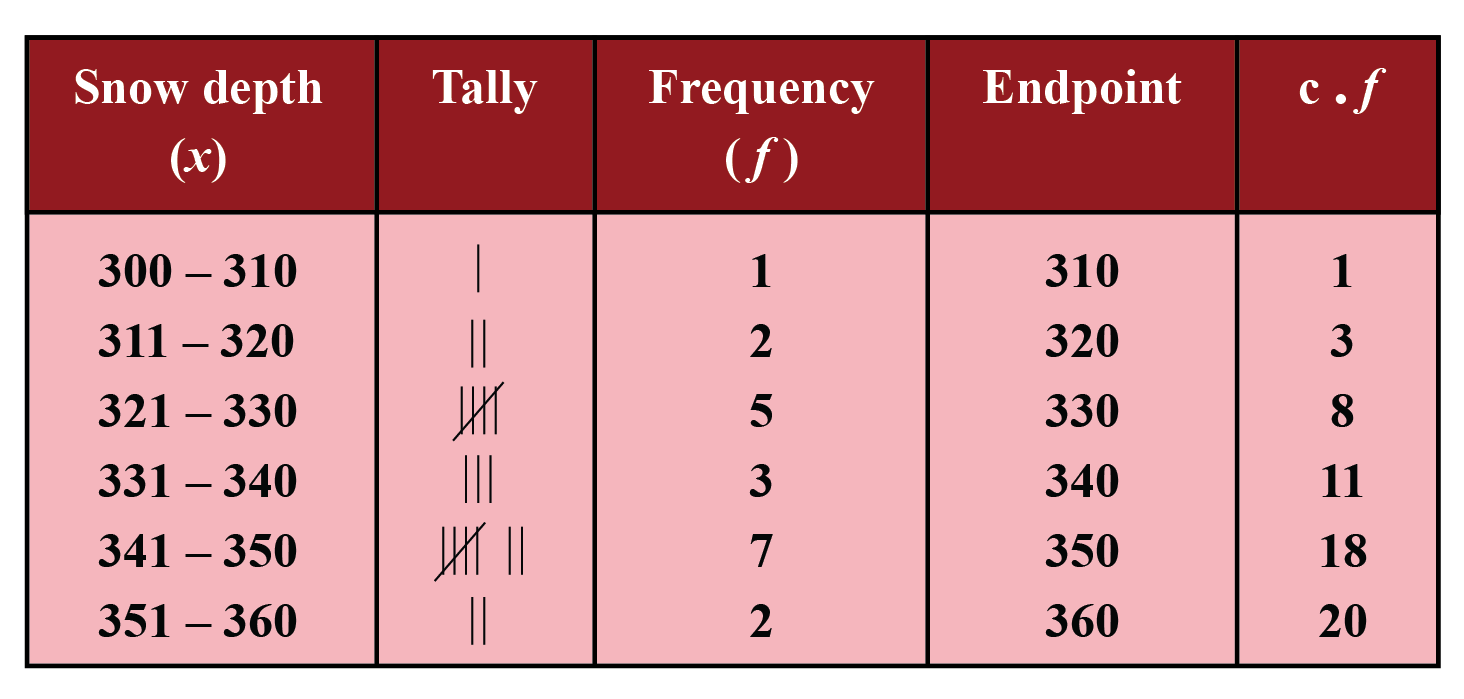Given measurements of snow depths are: 301, 312, 319, 354, 359, 345, 348, 341, 347, 344, 349, 350, 325,323, 324, 328,322, 332, 334, 337

Step 1: The snow depth measurements range from 301 cm to 359 cm. To produce the frequency distribution table, the data can be grouped in class intervals of 10 cm each.

In the Snow depth column, each 10-cm class interval from 300 cm to 360 cm is listed.

Step 2: The frequency column will record the number of observations that fall within a particular interval. The tally column will represent the observations only in numerical form.

Step 3: The endpoint is the highest number in the interval, regardless of the actual value of each observation.

For example, in the class interval of 311-320, the actual value of the two observations is 312 and 319. But, instead of using 219, the endpoint of 320 is used.

Step 4: The cumulative frequency column lists the total of each frequency added to its predecessor.

There are two types of cumulative frequency distributions
(1) Less than type cumulative frequency

(2) Greater than type cumulative frequency

### Less than type c.f.

It is obtained by adding successively the frequencies of all the previous classes including the class against which it is written. The cumulate is started from the lowest to the highest size.

### Greater than type c.f.

It is obtained by finding the cumulative total of frequencies starting from the highest to the lowest class. It is also called more than type cumulative frequency.

Example: Write down less than type cumulative frequency and greater than type cumulative frequency for the following data.

Height  (in cm)    Frequency (students)
140 – 145                          2
145 – 150                          5
150 – 155                          3
155 – 160                          4
160 – 165                          1

Solution:  We would have 'less than type' and 'more than type' frequencies as: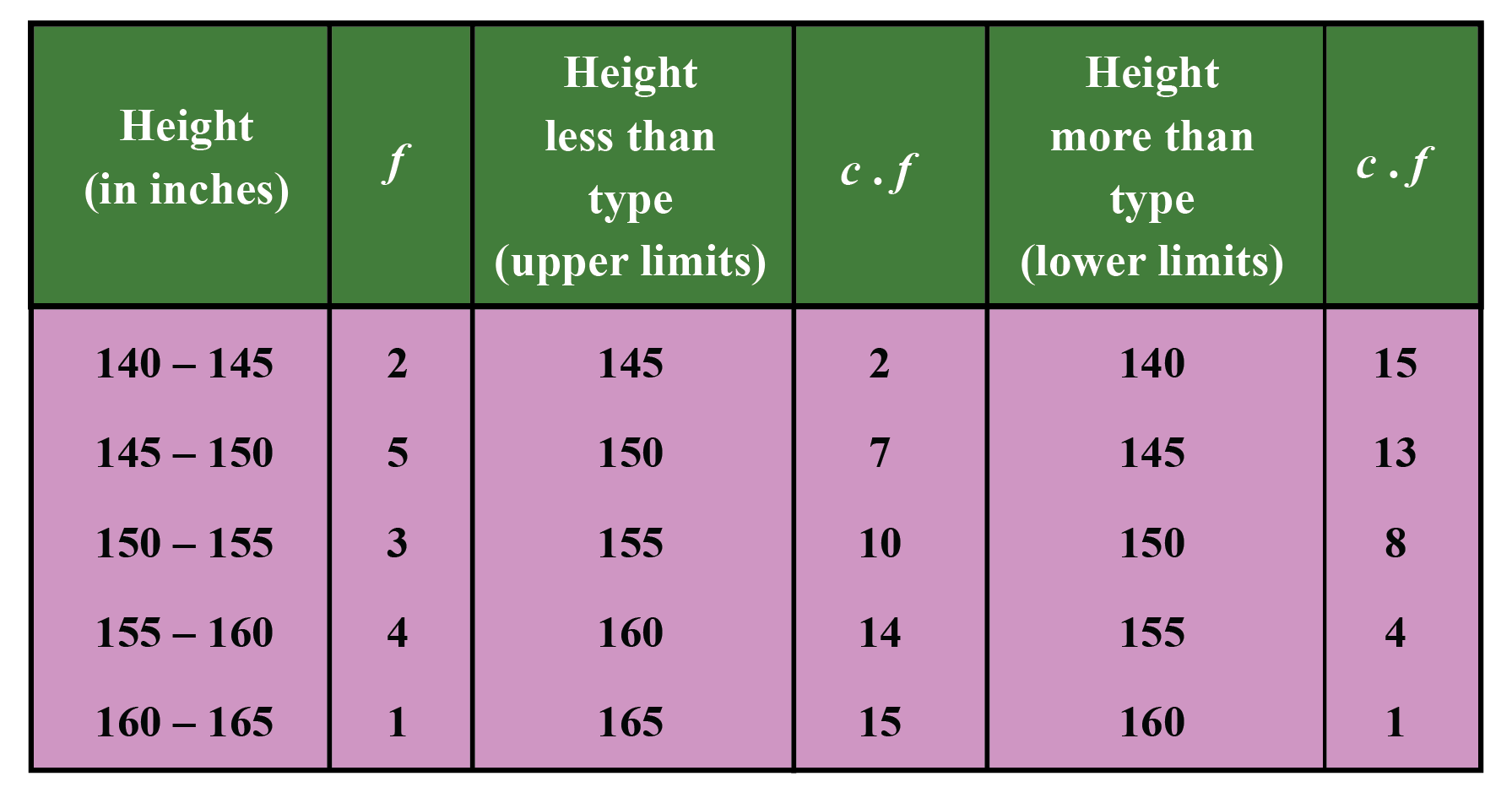The following information can be gained from either graph or table

• Out of a total of 15 students, 8 students have a height of more than 150 cm
• None of the students are taller than 165 cm
• Only one of the 15 students has a height of more than 160 cm

## Cumulative Frequency Calculator

Here's the activity on calculating the 'less than type' and 'more than type' cumulative frequency.

From this, select the type of frequency then tap/click on the given intervals for calculating both types of cumulative frequencies.

It will show you and help you visualize the frequencies considered while calculating the cumulative frequencies of both types for that particular interval.

## How to Make a Cumulative Frequency Distribution Graph?

A picture often makes it easier to understand things than just plain text.

In the world of statistics, graphs, in particular, are very important, as they help us to visualize the data and understand it better. So let us learn about the graphical representation of the cumulative frequency.

Cumulative frequency distribution of grouped data can be represented on a graph. Such a representative graph is called a cumulative frequency curve or an ogive.

There are two types of Cumulative Frequency Curves (or Ogives) :

Type 1: More than type Cumulative Frequency Curve

Type 2: Less than type Cumulative Frequency Curve

Steps to make a cumulative frequency distribution graph or Ogive are:

Steps How to plot a More than type Ogive? How to plot a Less than type Ogive?
1 Mark the lower limit on the x-axis Mark the upper limit on the x-axis
2 Mark the cumulative frequency on the y-axis. Mark the cumulative frequency on the y-axis.
3 Plot the points (x,y) using lower limits (x) and their corresponding Cumulative frequency (y) Plot the points (x,y) using upper limits (x) and their corresponding Cumulative frequency (y)
4 Join the points by a smooth freehand curve.       Join the points by a smooth freehand curve.

Example: Graph the two ogives for the following frequency distribution of the weekly wages of the given number of workers.

Weekly wages No. of workers
0-20 4
20-40 5
40-60 6
60-80 3

Solution:

Weekly wages No. of workers C.F. (Less than) C.F. (More than)
0-20 4 4 18
20-40 5 9 14
40-60 6 15 9
60-80 3 18 3

Less than curve:
Mark the upper limits of class intervals on the x-axis and take the less than type cumulative frequencies on the y-axis.

For plotting less than type curve, points (20,4), (40,9), (60,15), and (80,18) are plotted on the graph and these are joined by freehand to obtain the less than ogive.

Greater than ogive:

Mark the lower limits of class intervals on the x-axis and take the greater than type cumulative frequencies on the y-axis.

For plotting greater than type curve, points (0,18), (20,14), (40,9), and (60,3) are plotted on the graph and these are joined by freehand to obtain the greater than type ogive.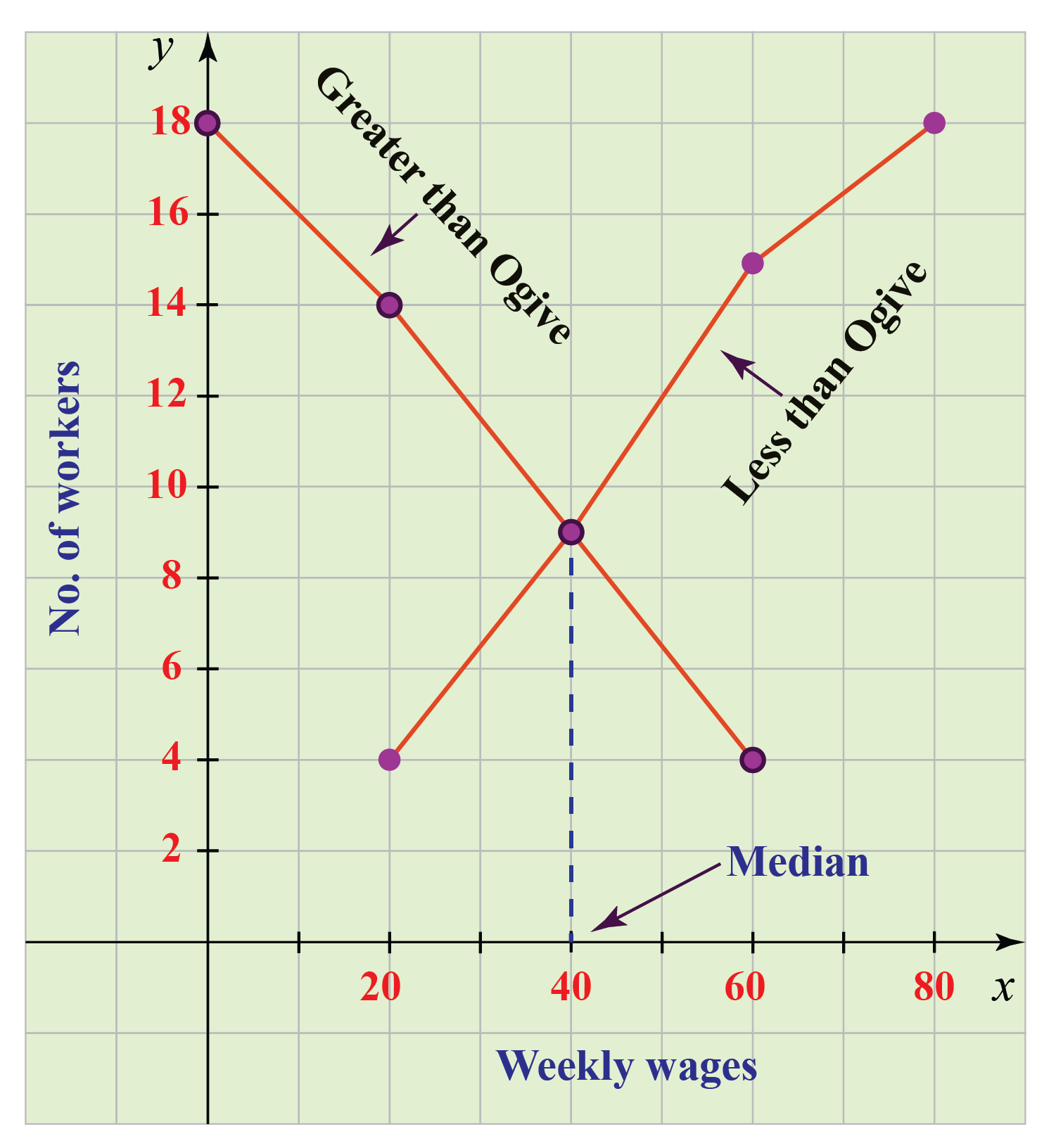A perpendicular line on the x-axis is drawn from the point of intersection of these curves. This perpendicular line meets the x-axis at a certain point, this determines the median. Here the median is 40.

The median of the given data could also be found from cumulative graphs. On drawing both the curves on the same graph, the point at which they intersect, the corresponding value on the x-axis, represents the Median of the given data set.

## Relative Cumulative Frequency

A car dealer wants to calculate the total sales for the past month and wants to know the monthly sales in percentage after weeks 1, 2, 3, and 4. Create a relative cumulative frequency table and present the information that the dealer needs.

Week No. of Cars Sold
1 10
2 17
3 14
4 11

First total up the sales for the entire month:

10 + 17 + 14 + 11 = 52 cars

Then find the relative frequencies for each week by dividing the number of cars sold that week by the total:

• The relative frequency for the first week is: 10/52 = 0.19
• The relative frequency for the second week is: 17/52 = 0.33
• The relative frequency for the third week is: 14/52 = 0.27
• The relative frequency for the fourth week is: 11/52 = 0.21

To find the relative cumulative frequencies, start with the frequency for week 1, and for each successive week, total all of the previous frequencies

Week Cars Sold Relative Frequency Cumulative Frequency
1 10 0.19 0.19
2 17 0.33 0.19 + 0.33 = 0.52
3 14 0.27 0.52 + 0.27 = 0.79
4 11 0.21 0.79 + 0.21 = 1

Note that the first relative cumulative frequency is always the same as the first relative frequency, and the last relative cumulative frequency is always equal to 1.

## Solved Examples

 Example 1

Create a cumulative frequency table showing the number of hours per week that Ryan plays video games, based on the given information.Ryan's Game Time

Monday: 2 hrs
Tuesday: 1 hr
Wednesday: 2 hrs
Thursday: 3 hrs
Friday: 4 hrs
Saturday: 2 hrs
Sunday: 1 hr

Solution

A cumulative frequency table for Ryan's game time can be made as follows:

Day Frequency (Hours) Cumulative Frequency (Hours)
Monday 2 2
Tuesday 1 2 + 1 = 3
Wednesday 2 3 + 2 = 5
Thursday 3 5 + 3 = 8
Friday 4 8 + 4 = 12
Saturday 2 12 + 2 = 14
Sunday 1 14 + 1 = 15

Thus, Ryan spends 15 hours of gaming in a week.

 $$\therefore$$ Ryan spends $$15$$ hours gaming in a week.
 Example 2

A weather forecaster highlights the lows over-night for the past 10 days in a small town in Wisconsin. The temperature readings are given in degrees Fahrenheit and are shown below. Use the data to make a frequency table.

41, 58, 41, 54, 49, 46, 52, 53, 55, 52

Solution

Frequency is nothing but the number of times an event occurs in a given scenario.

We will first choose a suitable class interval for the above data, then we will enter the frequency values to complete the table.

Interval Frequency
40-44 2
45-49 2
50-54 4
55-59 2
 Example 3

20 students were asked about the number of countries they have visited. The results are listed below. Complete the frequency table using this data.

6, 11, 4, 5, 0, 7, 1, 5, 4, 4, 3, 2, 2, 3, 2, 4, 3, 13, 0, 7

Solution

Interval Frequency  Cumulative Frequency
0-2 6 6
3-5 9 6 + 9 = 15
6-8 3 15 + 3 = 18
9-11 1 18 + 1 = 19
12-14 1 19 + 1 = 20
 Example 4

The following graph shows the participants in Jacob’s guitar classes. How many total students does he teach on the given day?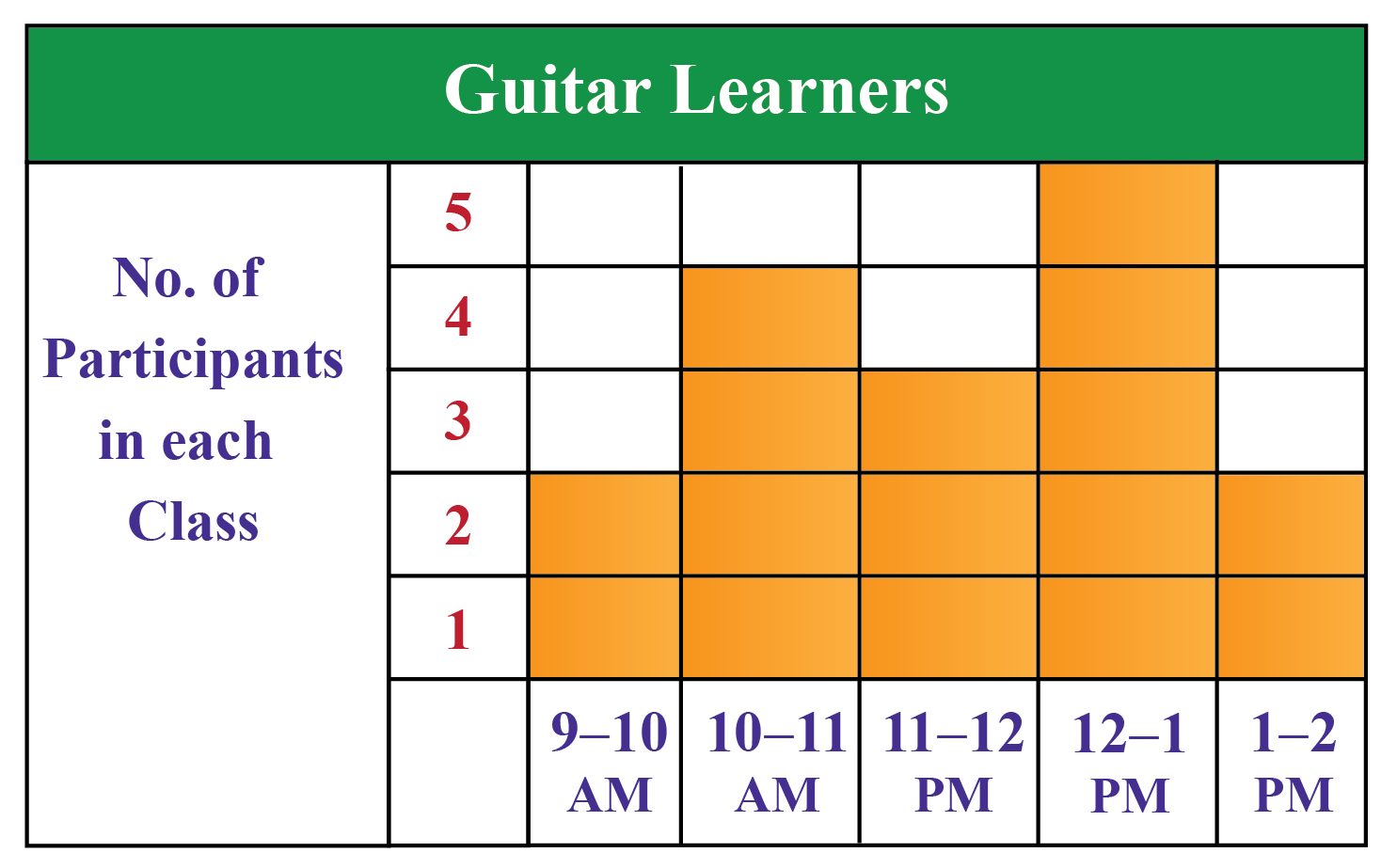Solution

Total students taught by Jacob = 2 + 4 + 3 + 5 + 2 = 16 students

 $$\therefore$$ Jacob teaches $$16$$ students in a day
 Example 5

The following represents scores that a class of 20 students received on their most recent Biology test. Plot a less than type Ogive.

58, 79, 81, 99, 68, 92, 76, 84, 53, 57, 81, 91, 77, 50, 65, 57, 51, 72, 84, 89

Solution

The cumulative frequency distribution table can be created as:

Interval Frequency  Less than type Cumulative Frequency
50-59 6 6
60-69 2 6 + 2 = 8
70-79 4 8 + 4 = 12
80-89 5 12 + 5 = 17
90-99 3 17 + 3 = 20

For plotting a less than type ogive the steps are given below:

1. Mark the upper limit on the x-axis.
2. Mark the cumulative frequency on the y-axis.
3. Plot the points (x,y) using upper limits (x) and their corresponding cumulative frequency (y).
4. Join the points using a freehand curve.## Interactive Questions

Here are a few activities for you to practice.

## Let's Summarize

We hope you enjoyed learning about the cumulative frequency with the simulations, cumulative frequency calculator, and practice questions. Now you will be able to easily solve problems on relative cumulative frequency, cumulative frequency table, and cumulative frequency graph.

At Cuemath, our team of math experts is dedicated to making learning fun for our favorite readers, the students!

Through an interactive and engaging learning-teaching-learning approach, the teachers explore all angles of a topic.

Be it worksheets, online classes, doubt sessions, or any other form of relation, it’s the logical thinking and smart learning approach that we, at Cuemath, believe in.

## 1. How do you calculate cumulative frequency?

In statistics, the frequency of the first-class interval is added to the frequency of the second class, and this sum is added to the third class and so on then, frequencies which are obtained this way are known as cumulative frequency (c.f.).

## 2. How many types of cumulative frequency are there?

There are two types of cumulative frequencies.

Type 1: Less than cumulative frequency

Type 2: More than cumulative frequency

## 3. How do you solve for more than cumulative frequency?

It is obtained by finding the cumulative total of frequencies starting from the highest to the lowest class. It is also called more than type cumulative frequency.

## 4. What is a cumulative series?

Cumulative frequency series is the series of frequencies which are continuously added corresponding to each class interval.

## 5. How do you plot cumulative frequency?

A cumulative frequency diagram is drawn by plotting the upper-class/ lower-class boundary with the cumulative frequency.

Cumulative frequency is plotted on the vertical axis and class boundaries are plotted on the horizontal axis.

Steps to make a cumulative frequency graph are:

1. Mark the class limit on the x-axis.
2. Mark the cumulative frequencies on the y-axis.
3. Plot the points (x,y) using the class limit (x) and their corresponding cumulative frequency (y).
4. Join the points by a smooth freehand curve.
More Important Topics
Numbers
Algebra
Geometry
Measurement
Money
Data
Trigonometry
Calculus